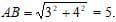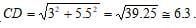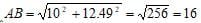# Test: Quantitative Aptitude- 1

## 30 Questions MCQ Test Quantitative Aptitude for GMAT | Test: Quantitative Aptitude- 1

Description
Attempt Test: Quantitative Aptitude- 1 | 30 questions in 60 minutes | Mock test for GMAT preparation | Free important questions MCQ to study Quantitative Aptitude for GMAT for GMAT Exam | Download free PDF with solutions
QUESTION: 1

### 15 Java programmers, working in a constant pace, finish a web page in 3 days. If after one day, 9 programmers quit, how many more days are needed to finish the remainder of the job?

Solution:

The total working days for finishing a web page are (15 x 3) 45. If after one day 9 programmers quit, only 15 working days are done and the rest of the programmers (6)

Need to finish (45 – 15) 30 days of work. It will take them 5 more days.

QUESTION: 2

### Two carpenters, working in the same pace, can build 2 desks in two hours and a half. How many desks can 4 carpenters build in 4 hours?

Solution:

⇒ 2 carpenters build 2 desks in 2.5 hours

⇒ 4 carpenters build 4 desks in 2.5 hours

⇒ In 4 hours there are (4/2.5 = 1.6) time units. And (4 x 1.6) is 6.4 desks.

QUESTION: 3

### There are 40 students in a classroom, 9/20 of them are boys and 4/5 of them are right-handed. How many right-handed boys are there in the classroom?

Solution:

Given that there are 18 boys (9/20*40=18) and 22 girls in the class. Also we know that out of 40 students 32 are right-handed (4/5*40=32).

Maximum number of right-handed boys possible is if ALL 18 boys are right-handed;
Minimum number of right-handed boys possible is if ALL 22 girls are right-handed, so in this case 32-22=10 boys would be right-handed.

QUESTION: 4

In Jonathan’s pen there are 300 sheep’s. 5/6 of the sheep’s are white, 2/3 of the sheep’s have soft wool. What can’t be the number of white sheep’s that also have soft wool in the pen?​

Solution:

There are (5/6 x 300 = 250) white sheep’s.
There are (2/3 x 300 = 200) soft woolen sheep’s.
The maximum overlap is the size of the smallest among the groups, thus 200. The minimum overlap is (250 + 200 – 300 = 150).
Therefore the number of sheep’s can be somewhere between 150 and 200.

QUESTION: 5

Ross has 40 shirts, ¾ of the shirts are green and 1/10 is without buttons.

Therefore Ross has between ___ and ___ shirts with buttons that are not green.

Solution:

Notice that the groups that we are looking for a overlapping are the not-green shirts and the buttoned ones. The not-green shirts are a quarter of 40, 10 shirts.
The shirts with buttons are (9/10 x 40 = 36).
The maximum overlapping is the size of the smallest group: 10.
The minimum overlapping is: 36 + 10 – 40 = 6.

QUESTION: 6

In the Kan film festival, 50 movies were presented. 3/5 of the movies are action movies and 4/5 is science fiction movies. How many of the movies were science fiction action movies?

Solution:

There were (3/5 x 50 = 30) action movies.
There were (4/5 x 50 = 40) science fiction movies.
Exact overlapping is calculated by minimum overlapping method.
Therefore there are (40 + 30 – 50 = 20) movies that belong to both categories.

QUESTION: 7

There are 200 cats in Cat-City. Out of the 200, 70 are street cats and the rest are domestic cats. 110 cats are gray, 30 out of the gray cats are street ones. How many domestic cats are there which are not gray in Cat-City?

Solution:

Out of 200 cats, 130 are domestic ones. Out of 110 gray cats, 30 are street cats therefore 80 are grey and domestic ones.
Altogether there are 130 domestic cats, 80 are grey so (130 – 80) = 50 are not grey.

QUESTION: 8

Chandler is building a fence in the following method: He grounds 10 poles, each 10 Cm thick, in 1 meter spaces from each other. He then connects the poles with a barbed wire. What is the total length of the fence?

Solution:

The total width of the poles is (10 x 0.1 = 1) meter.
There are 9 spaces between the poles, each 1 meter, so it’s another 9 meters.
The total length is (1 + 9 = 10) meters.

QUESTION: 9

In a psychology school the grade of the students is determined by the following method: At the end of the first year the grade equals to twice the age of the student. From then on, the grade is determined by twice the age of the student plus half of his grade from the previous year. If Joey’s grade at the end of the first year is 40, what will be his grade at the end of the third year?

Solution:

From the grade 40 at the end of the first year we learn that his age is 20.
At the end of the second year, he will be 21 and his grade will be
(21 x 2 + ½ x 40 = 62).
At the end of the third year, he will be 22 and his grade will be (22 x 2 + ½ x 62 = 75).

QUESTION: 10

What is the sum of all the even numbers bigger than (-10) and smaller than 12?

Solution:

This is a series of numbers with a constant spacing between them.
The first number is (-8) and the last is (10), there are 10 numbers altogether.
The formula for such a series is: ((-8 + 10) x 10)/2 = 10.
The second way to answer such a question is to write the numbers and add them

QUESTION: 11

The value of an “Aerosoul” stock changes according to the following method:At the end of each month her value is doubled but due to commission the stock’s value is decreases by \$10. If the value at the beginning of January is \$A, what would be her value at the end of February?

Solution:

At the end of January her value is 2A – 10.
At the end of February her value is (2 x (2A – 10) – 10 = 4A – 30).

QUESTION: 12

An Ameba is an organic life form that divides into two Amebas each round hour. If at a certain round hour, two Amebas were placed in a jar, how many Amebas will be in the jar in N hours?

Solution:

Let’s find the number of Amebas in the first hours.
After one hour (N=1) there will be 4 Amebas.
After two hours (N=2) there will be 8 Amebas.
After three hours (N=3) there will be 16 amebas.
Therefore the formula that fits this series is 2N+1.

QUESTION: 13

Alfa, Beta and Gamma are inner angles in a triangle. If Alfa = Beta + Gamma, what can’t be the size of Beta?

Solution:

If Beta is 90 degrees than Alfa is bigger than 90 and the sum of the angles in the triangle will be bigger than 180 degrees.

QUESTION: 14

In a triangle, one side is 6 Cm and another side is 9 Cm. which of the following can be the perimeter of the triangle?

Solution:

The third side of the triangle is larger than 3 (The difference between the other two) and smaller than 15 ( The sum of the other two).
The perimeter is between (6+9+3 = 18) and (6+9+15 = 30). The only answer that is in this range is B.

QUESTION: 15

To which of the following shapes the area can’t be calculated if the perimeter is given?

Solution:

The perimeter of a rectangle is 2a + 2b. In order to calculate the area we need to know the multiplication of a x b.

QUESTION: 16

A and B are two circles. The radius of A is twice as large as the diameter of B. What is the ratio between the areas of the circles?

Solution:

The radius of circle A is 4 times larger than the radius of circle B. The area of a circle is a function of the radius squared, therefore the area of radius A is 16 times bigger.

QUESTION: 17

A, B, C, D and E are 5 consecutive points on a straight line. If BC = 2CD, DE = 4, AB = 5 and AC = 11, what is the length of AE?

Solution:

First, draw the line and the points.
In order to find the length of AE, find the length of CD and BC first.
BC = AC – AB = 11 – 5 = 6.
BC = 2CD à CD = 3.
AE = 5 + 6 + 3 + 4 = 18.

QUESTION: 18

In a rectangular axis system, what is the distance between the followingpoints: A(3,2) and B(7,5) ?

Solution:

First, draw a rectangular axis system and mark the two points.
The easiest way to find the distance between them is to draw a triangle, where the line AB is the hypotenuse. You can see that the length of one side of the triangle is (5-2=3) and the other side is (7-3=4). The length of the line AB is received with the help Of the Pythagoras principle:QUESTION: 19

In a rectangular axis system, what is approximate distance between the following  points: C(1,2.5) and D(6.5,5.5) ?

Solution:

First, draw a rectangular axis system and mark the two points.
The easiest way to find the distance between them is to draw a triangle, where the line CD is the hypotenuse. You can see that the length of one side of the triangle is (5.5 - 2.5 = 3) and the other side is (6.5 – 1 = 5.5). The length of the line CD is received with the help Of the Pythagoras principle:QUESTION: 20

In a rectangular axis system, what is the distance between the following points: A(24.4,30) and B(34.4,42.49) ?

Solution:

First, draw a rectangular axis system and mark the two points.

The easiest way to find the distance between them is to draw a triangle, where the line AB is the hypotenuse. You can see that the length of one side of the triangle is

(34.4 – 24.4 = 10) and the other side is (42.49 – 30 = 12.49). The length of the line AB is received with the help of the Pythagoras principle:QUESTION: 21

In a rectangular axis system, what is the area of a parallelogram with the coordinates: (5,7), (12,7), (2,3), (9,3) ?

Solution:

First, draw the axis system and mark the 4 points. Connect the points to get a parallelogram. The area is calculated by the multiplication of one on of the bases and the height. The height is (7 – 3 = 4), the length of the base is (9 – 2 = 7).
The area is 4 x 7 = 28.

QUESTION: 22

If the radius of a cylinder is doubled and so is the height, what is the new volume of the cylinder divided by the old one?

Solution:

The volume of a cylinder is (pie x R2) x (height of cylinder).
The new volume is (4 x 2 = 8) bigger.

QUESTION: 23

If the radius of a cylinder is doubled and so is the height, how much bigger is the new lateral surface area (with out the bases)?

Solution:

The lateral surface area of a cylinder is (2 x pie x R) x (height of cylinder).
The new lateral surface area is (2 x pie x 2R) x (double the height) = 4 times bigger.

QUESTION: 24

If  X ~ Y = X2 + XY, then what is the value of  -1 ~ 2 ?

Solution:

The best answer is B.-1 ~ 2 = (-1)2 + (-1)2 = -1.

QUESTION: 25

For every X, the action [X] is defined in the following matter: [X] is the greatest integer that is smaller or equal to X. For example: [8.9] = 8.

What is the value of [6.5] x [2/3] +  x 7.2 + [8.4] – 6.6 ?

Solution:

[6.5] x [2/3] +  x 7.2 + [8.4] – 6.6 = 6 x 0 + 2 x 7.2 + 8 - 6.6 = 15.8.

QUESTION: 26

If  (1 < A < 3 < B), then which of the following expressions is the largest?

Solution:

Try some numbers and check the answers. A=2, B=4.
(a) 6/1 = 6
(b) 2/3
(c) 1/2
(d) 2
(e) 2

QUESTION: 27

Which of the following fractions is the smallest?

Solution:

.Compare all of the answers to
(a) 3/10.
(b) 3/10 x 2 = 6/20 which is smaller than 6/19.
(c) 3/10 is smaller.
(d) 3/10 = 9/30, and this is smaller than 11/30.
(e) 3/10 = 12/40 and that is smaller than 12/31.
The smallest fraction is A.

QUESTION: 28

Which of the following fractions is the largest?

Solution:

Lets compare all the answers to 2/7, unless we find a larger fraction.
(b) 2/3 is larger than 2/7. For now, this is the right answer.
(c)  2/3 is also 6/9 and that is smaller than 7/9. For now this is the right answer.
(d) 7/9 is bigger than 7/12
​(e) Bring this answer and (c) to a common denominator.7/9 = 35/45  and  3/5 = 27/45.
7/9 is the largest fraction.

QUESTION: 29

If A2 + B2 = 15 and AB = 10, what is the value of the expression (A – B)2  + (A + B)2 ?

Solution:Use Code STAYHOME200 and get INR 200 additional OFF Use Coupon Code